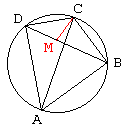# Ptolemy's Theorem

Let a convex quadrilateral $ABCD$ be inscribed in a circle. Then the sum of the products of the two pairs of opposite sides equals the product of its two diagonals. In other words,

$AD\cdot BC + AB\cdot CD = AC\cdot BD.$

## Remark

Ptolemy of Alexandria (~100-168) gave the name to the Ptolemy's Planetary theory which he described in his treatise Almagest. The book is mostly devoted to astronomy and trigonometry where, among many other things, he also gives the approximate value of $\pi$ as $377/120$ and proves the theorem that now bears his name. The name Almagest is actually a corruption of the Arabic rendition "Al Magiste" - The Greatest - of the Greek H Megisth Suntaxiz (E Megiste Syntaxis).This classical theorem has been proved many times over. Following is the simplest proof I am aware of. (There is another simple proof of a recent vintage.)

## Proof

On the diagonal $BD$ locate a point $M$ such that angles $ACB$ and $MCD$ be equal. Since angles $BAC$ and $BDC$ subtend the same arc, they are equal. Therefore, triangles $ABC$ and $DMC$ are similar. Thus we get $CD/MD = AC/AB,$ or $AB\cdot CD = AC\cdot MD.$

Now, angles $BCM$ and $ACD$ are also equal; so triangles $BCM$ and $ACD$ are similar which leads to $BC/BM = AC/AD,$ or $BC\cdot AD = AC\cdot BM.$ Summing up the two identities we obtain

$AB\cdot CD + BC\cdot AD = AC\cdot MD + AC\cdot BM = AC\cdot BD$

## Remark

Ptolemy's theorem admits a useful generalization: for four points $A,$ $B,$ $C,$ $D,$ not necessarily concyclic,

$AB\cdot CD + BC\cdot AD \ge AC\cdot BD$

which is known as Ptolemy's inequality.The following problem is discussed in Honsberger, Mathematical Morsels, p172:Let $A_{1}A_{2}A_{3}$ denote an equilateral triangle inscribed in a circle. For any point $P$ on the circle, show that the two shorter segments among $PA_{1},$ $PA_{2},$ $PA_{3}$ add up to the third one.

## Solution

Let s denote the length of the side of the given triangle. By Ptolemey's Theorem we have

$s\cdot PA_{1}=s\cdot PA_{2}+s\cdot PA_{3}$

Therefore,

$PA_{1}=PA_{2}+PA_{3}.$

### Remark

This result has an interesting generalization to the case of a regular $3n$-gon inscribed in a circle: Of the 3n chords obtained by connecting a point $P$ with vertices of the polygon, the sum of the $2n$ shortest ones equals the sum of the n longest.

The problem itself is sometimes attributed to Van Schooten (1615 - 1660), see, for example, The Changing Shape of Geometry, (C. Pritchard, Cambridge University Press, 2003), p. 184, where two additional proofs could be found.

### Remark

Ptolemy's theorem is a powerful result. With its help we establish the Pythagorean Theorem and

• Carnot's Theorem. Combined with the Law of Sines, Ptolemy's theorem serves to prove the addition and subtraction formulas for the sine function. It has a short proof in complex numbers. The following generalization is sometimes attributed to the great $9^{th}$ century Indian mathematician Mahavira (or Mahaviracharya, meaning Mahavira the Teacher). However, the formulas derived below have been already known to the $7^{th}$ century Indian mathematician Brahmagupta.

In a cyclic quadrilateral $ABCD,$ let $a,$ $b,$ $c,$ $d$ denote the lengths of sides $AB,$ $BC,$ $CD,$ $DA,$ and $m,$ $n$ the lengths of the diagonals $BD$ and $BC.$ Then Mahavira's result is expressed as

$\displaystyle m^{2} = \frac{(ab + cd)(ac + bd)}{ad + bc}$ and
$\displaystyle n^{2} = \frac{(ac + bd)(ad + bc)}{ab + cd}.$

H. Eves gives a proof as a sequence of exercises in [Great Moments in Mathematics Before 1650, p. 108]:

Let $t$ be the diameter of the circmcircle of $ABCD$ and $\theta$ the angle between either diagonal and the perpendicular upon the other.

Then, (using triangle's formula $ab = 2hR$ applied to $DAB$ and $DCB),$ we get

$mt\cdot \cos \theta = ab + cd$ and
$nt\cdot \cos \theta = ad + bc.$

So $\displaystyle\frac{m}{n} = \frac{ab + cd}{ad + bc}$ which is called Ptolemy's second theorem.

Also $mn = ac + bd$ (Ptolemy relation)

Multiplying those last 2 equations, we get:

$\displaystyle m^{2} = \frac{(ab + cd)(ac + bd)}{ad + bc}.$

$\displaystyle n^{2} = \frac{(ac + bd)(ad + bc)}{ab + cd}.$

Finally, we also get

$\displaystyle (t \cos \theta )^{2} = \frac{(ab + cd)(ad + bc)}{ac + bd}.$

And if the diagonals in the quadrilateral are orthogonal,

$\displaystyle t^{2} = \frac{(ab + cd)(ad + bc)}{ac + bd}.$

An additional derivation has been posted to the old CTK Exchange. This one can be found in Advanced Trigonomentry by C. V. Durrell and A. Robson, 1930, p. 25. (The book is available in a 2003 Dover edition and on google's bookshelf.)

In the same notations as above, by the Cosine Rule

(1)

$m^{2}= b^{2} + c^{2} - 2\cdot bc\cdot \cos (C).$

And also

(2)

\begin{align} m^{2} &= a^{2} + d^{2} - 2\cdot ad\cdot \cos (A)\\ &= a^{2} + d^{2} - 2\cdot ad\cdot \cos (180^{\circ} - C)\\ &= a^{2} + d^{2} + 2\cdot ad\cdot \cos (C). \end{align}

Multiply (1) by ad and (2) by $bc,$ add them up and you will arrive at

$\displaystyle m^{2} = \frac{(ab + cd)(ac + bd)}{ad + bc}.$

The expression for $n^{2}$ is obtained by picking the other pair of triangles.### Ptolemy's Theorem